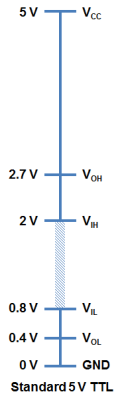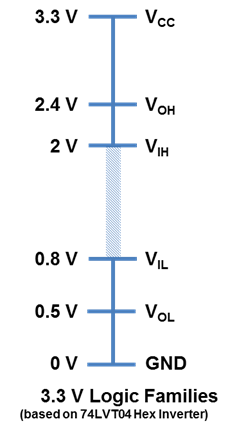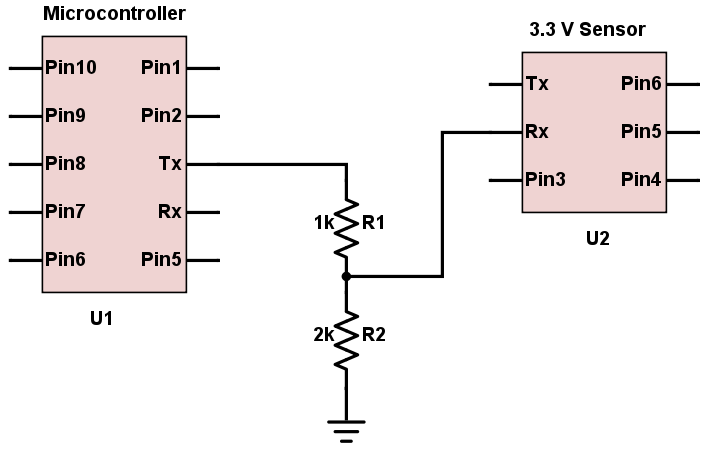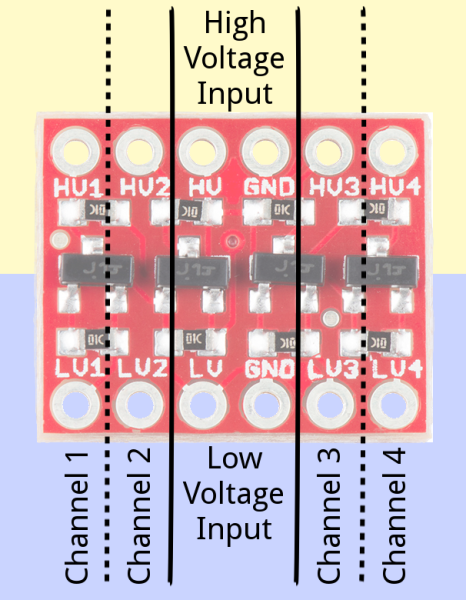# Logic Levels, Level Shifting and Voltage dividers - Arduino Concepts for Beginners

acfb Feb 04, 2020

## Logic Level

The concept of logic level is among the first you encounter when dealing with Arduino: like a switch that can be either ON or OFF, or binary 1 and 0 from boolean algebra, here we deal instead with a 2-state system of HIGH and LOW where the logic level is represented by the voltage difference between a signal and ground.

We speak thus of a LOW state, which broadly corrisponds to GND or 0V, and an HIGH state which usually is either 5V or 3.3V (the most common values in consumer electronics). Beware, though, that a device can be functioning with an active high logic - meaning it is enabled when you pass a "HIGH" state, or you connect the pin to Vcc - or a active low logic, where it performs its function when pin is "pulled" LOW by connecting it to ground.

As I said before, usually the LOW value is considered to be 0V while the HIGH value is either 5V or 3.3V. But why? Things are a bit complicated here, and it depends on the technology behind the device taken into consideration.
A TTL system (Transistor-Transistor-Logic, where bipolar junction transistors are used to create logic gates and so on) can generate a LOW signal at a minimum of 0.4V (V ol) and recognize an INPUT as LOW if it's up to 0.8V (V il). Conversely, a signal of 2V is the absolute minimum to consider an INPUT HIGH (V ih), while 2.7V is the minimum output signal considered HIGH by the device(V oh).
The following image, taken from the Sparkfun website, sums this up:That is, anything under 0.8V is LOW, anything over 2V is HIGH. But what about the inbetween values? That's where "undefined" behaviours arise and we call this state floating because electrical noise or nearby currents can make a pin float between HIGH and LOW in unpredictable ways.

Something similar to what we have seen for TTL systems exists for CMOS devices (Complementary Metal–Oxide–Semiconductor, uses complementary and symmetrical pairs of n- and p-type MOSFETs to create logic functions), but the scale has small differences: in the LOW state we have a minimum generated low (V ol) of 0.5V, while we have a minimum recognized HIGH at 2.4V. Since CMOS work with lower power they also operate at a lower voltage level, and that's why a Vcc of 3.3V is the top of the scale:When dealing with Arduino, though, we have a safer range of values, where the LOW is between 0.9V and 1.5V while the HIGH is between 3V and 5V, with 4.2V as minimum OUTPUT provided as HIGH.

## Level Shifting and Logic Level Converters (LLC)

Wheter a device works with a 5V or a 3.3V current depends on the technology used by the manufacturer, but the logic behind its workings is the same: HIGH or LOW. What will happen when we connect devices with different voltages for logic levels? We can have 2 situations:

• 3.3V device to a 5V device:
Usually there's no problem here, since a 5V device will still consider as HIGH a signal from the 3.3V device (anything down to 2V).

• 5V device connected to a 3.3V device:
Of course this situation can be very problematic: an Arduino Uno, for example, generates more than 4V for its HIGH state, and that's beyond any tolerance a 3.3V device can have (usually can be at most 3.6 / 3.7V).
We need to find a way to "convert" the HIGH state so that a device can understand and talk within that range.

On of the most basic circuit in electronics is the voltage divider (Wikipedia: Voltage Divider: just take a couple of resistors, add some maths (1) and you can reduce the voltage down to the value you require.With this in mind we can create a crude but easy "logic level shifter" that turns a 5v output into a 3.3V input:It's the cheapest and easiest way, but the cons are the unidirectionality of the flow (5V to 3.3V: ok, 3.3V to 5V: you need a more complex setup), the loss of precision (resistor tolerance, voltage fluctuations), power dissipation or parasitic capacitance of the circuit.

A better way it to use a Bi-direction logic level converter: the market is full of these cheap devices, they are very easy to setup (see image below, from the SparkFun website) and can be used not only for UART but also SPI and I2C communication.If you are curious to see what happens "under the hood" (basically it's a setup of MOSFETs and pullup resistors) you can check this awesome resource I found:

Bi-direction LLC on CircuitDigest 🔗

(1) Vout = Vin x R2/(R1 + R2)
Vout and Vin are what we know for sure, but what about resistor's values? We can pick any value for R1 and solve for the unknown member R2:
R2 = Vout * R1 / (Vin - Vout)